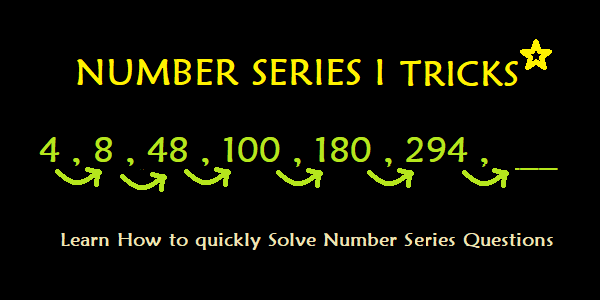# Number Series I – Number Series Tricks To Solve Questions in J&K Bank PO/BA Exam

In this post, we will discuss examples of questions from number series and solve those using number series tricks to save time in J&K Bank PO/BA Exam.

#### Number Series Tricks

Number Series Questions are numerical logical questions, frequently asked in J&K Bank PO/BA Exam. In number series, a sequence is given from which we have to deduce a pattern. Then that pattern is used to predict the missing number in the series. The difficulty level of number series questions can increase in two ways; the logic behind the sequence becomes less trivial, therefore demanding attention and creativity, or the missing number is positioned at an early stage where you can figure out the missing number by only looking at the previous numbers in the sequence. These number series questions are little tricky but they can easily be solved by using number series tricks.

Here, we have discussed few questions and have solved them using Number Series tricks to save time in J&K Bank PO/BA Exam.

Example 1: Questions solved using Number Series Tricks

### Question:  1, 2, 3, 4, 5, 6, ?

Solution:

To solve this number series question, we need to figure out the logic behind the series in order to find the missing number.

As

 1 2 3 4 5 6 ? 1 1+1 2+1 3+1 4+1 5+1 6+1 1 2 3 4 5 6 7

We can make out that every number is one more than the previous number.

Hence, 6 + 1 = 7

Therefore, 7 is the missing number.

Example 2: Questions solved using Number Series Tricks

Question:  2, 4, 6, 8, 10, 12, ?

Solution:

To solve this number series question we need to figure out the logic behind the series.

 2 4 6 8 10 12 ? 2 2+2 4+2 6+2 8+2 10+2 12+2 2 4 6 8 10 12 14

The numbers that are present in the series are all more than the previous number by  two.

Therefore, 14 is the missing number.

Example 3: Questions solved using Number Series Tricks

Question:  1, 2, 4, 7, 11, 16, ?

Solution: To solve this number series question we need to figure out the logic behind the series.

 1 2 4 7 11 16 ? 1 1+1 2+2 4+3 7+4 11+5 16+6 1 2 4 7 11 16 22

The difference between the numbers increase by 1 on each step.

Therefore, 22 is the missing number.

### Remain Updated with all the Notifications from Jk Bank. Like their Facebook Page below:

Example 4: Questions solved using Number Series Tricks

Question: 1, 4, 9, 16, 25, ?

Solution:

To solve this number series question we need to figure out the logic behind the series.

 1 4 9 16 25 ? 12 22 32 42 52 62 1 4 9 16 25 36

The numbers that are present in the series are all squares of integers with difference of one between them.

Therefore, 36 is the missing number.

Example 5: Questions solved using Number Series Tricks

Question:  2, 2, 12, 21, 22, ?

Solution:

To solve this number series question we need to figure out the logic behind the series. This one is a tricky series but you need to get any logic that satisfies the series. There can be interchanging of digits in a number. Let Interchanging be denoted by \$

 2 2 12 21 22 ? 2 \$(2) 2+10 \$(12) 12+10 \$(22) 2 2 12 21 22 22

The series starts with “2”. Since the second number is also “2”, we can conclude few possibilities:

1. Either we are raising power by 1 i.e., 21 = 2, but that does not explain the rest of the series.
2. Or, we are adding 0 to 2, still the remaining series remains a mystery.
3. Or, we are interchanging digits in the number. Since 2 will become 2 after interchanging digits. This also happens when 12 is followed by 21.

Now that we found, second term is reverse of first term, we need to find relation between first and the third term. If we take only first, third and fifth term, we will get the series:

 2 12 22 2 2+10 12+10

Number Series Questions can only be solved quickly if you keep on practicing.